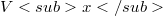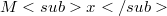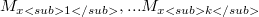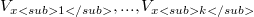﻿

### Maximal visibility and unions of orthogonally starshaped sets

#### Abstract

Let S be an orthogonal polygon in the plane. For each point x in S,let$Vx$ denote the set of points which x sees via staircase paths and let(Error rendering LaTeX formula). For S simply connected, S is starshaped via staircase paths (i.e., orthogonally starshaped) if and only if S contains exactly one such closed set$Mx$, and when this occurs$Mx$ is the staircase kernel of S. In general, if S contains exactly k such distinct closed set$M_{x1},...M_{xk}$, then S is a union of k (or possibly fewer) orthogonally starshaped sets chosen from$V_{x1},...,V_{xk}$.

DOI Code: 10.1285/i15900932v24n1p1

Keywords: Orthogonal polygons; Starshaped via staircase paths

Classification: 52A30

Full Text: PDF